Ex 6.3

Chapter 6 Class 12 Application of Derivatives
Serial order wise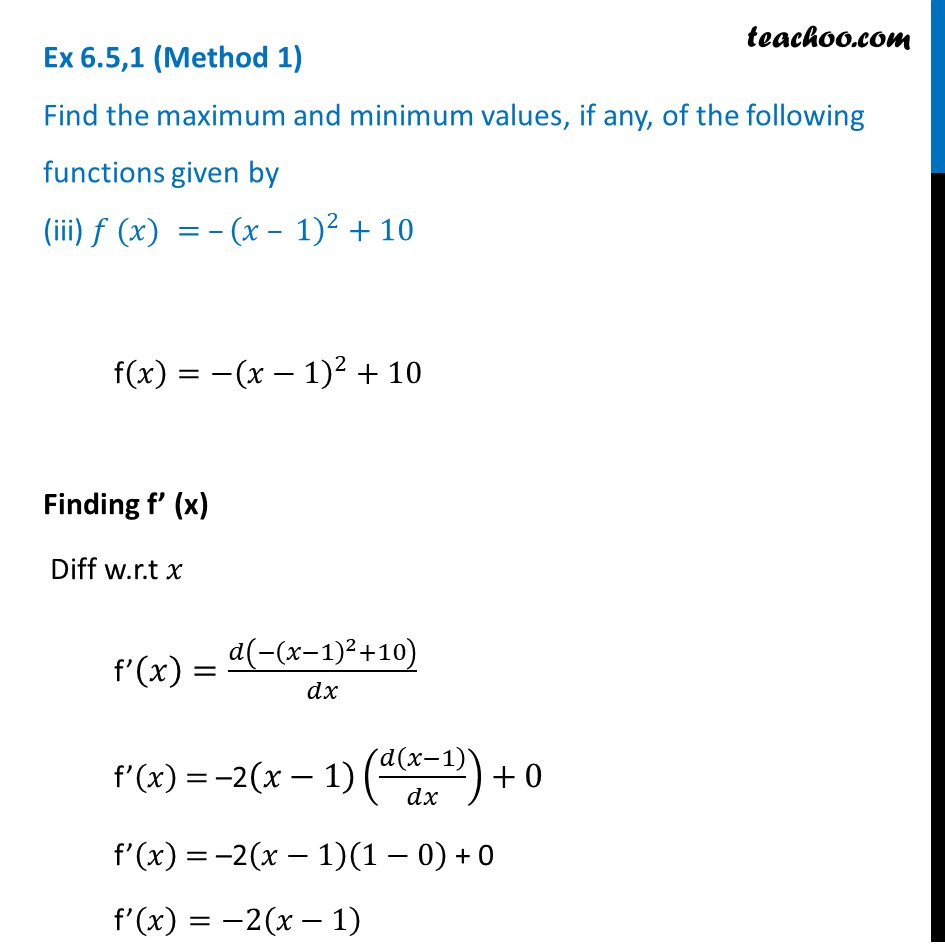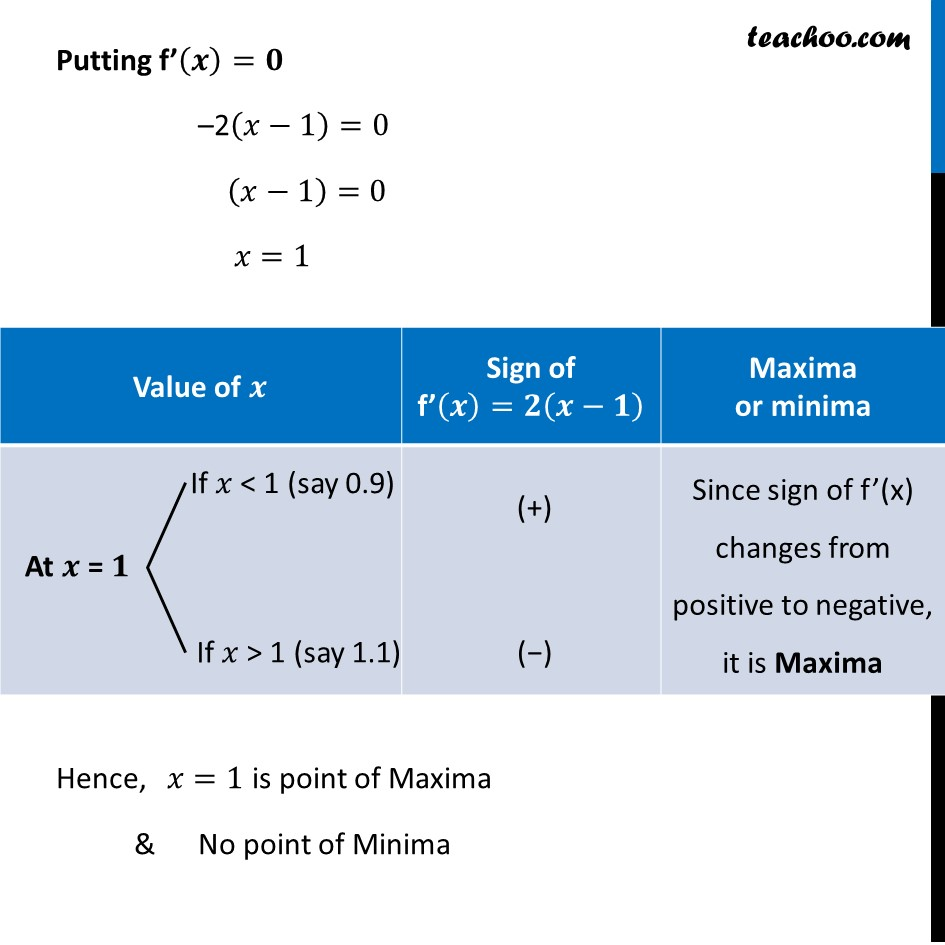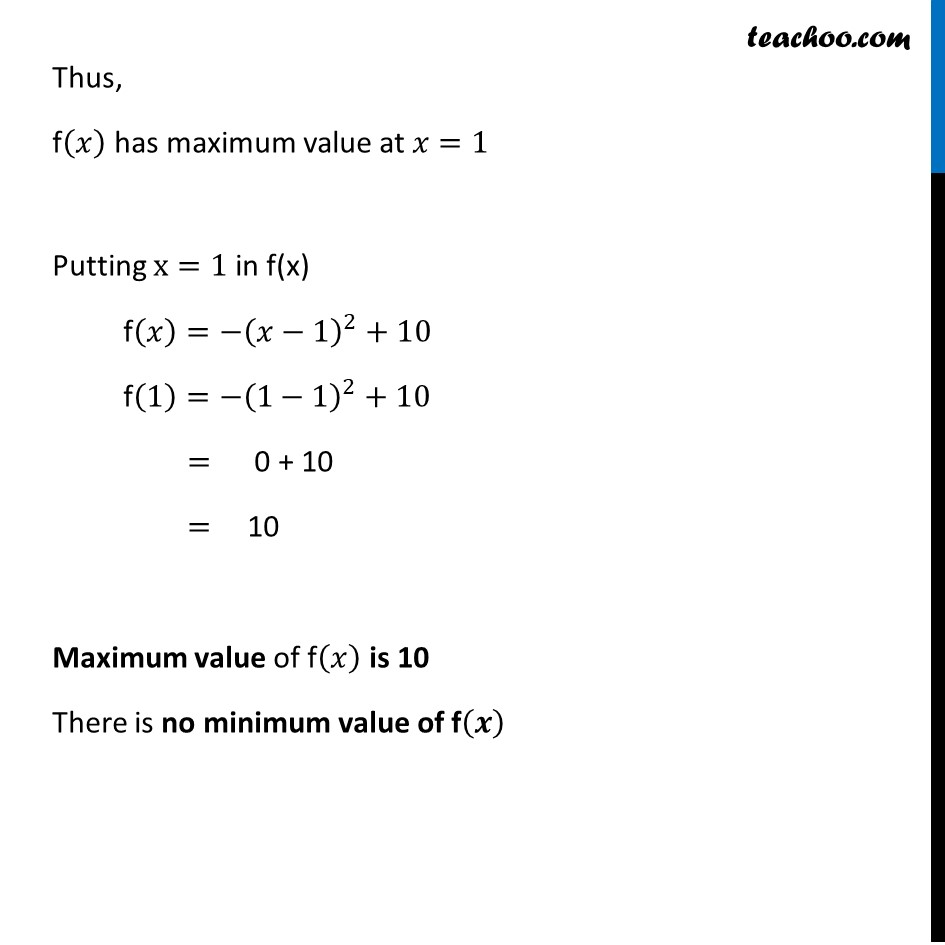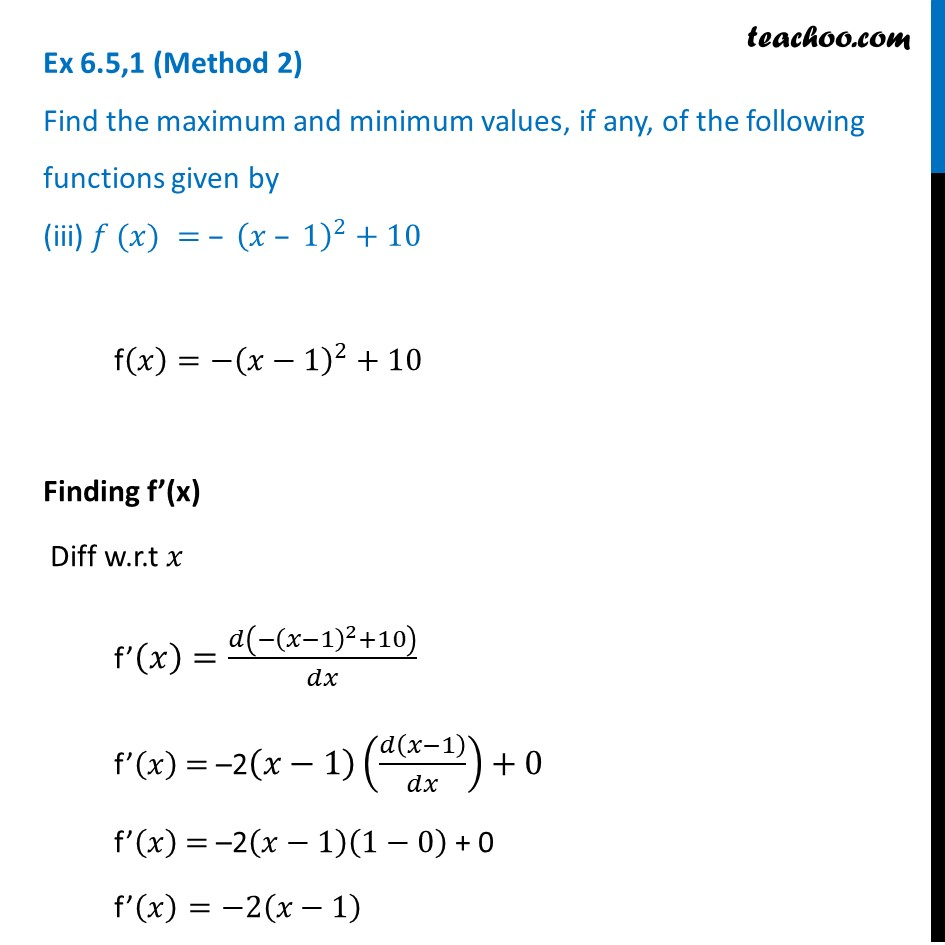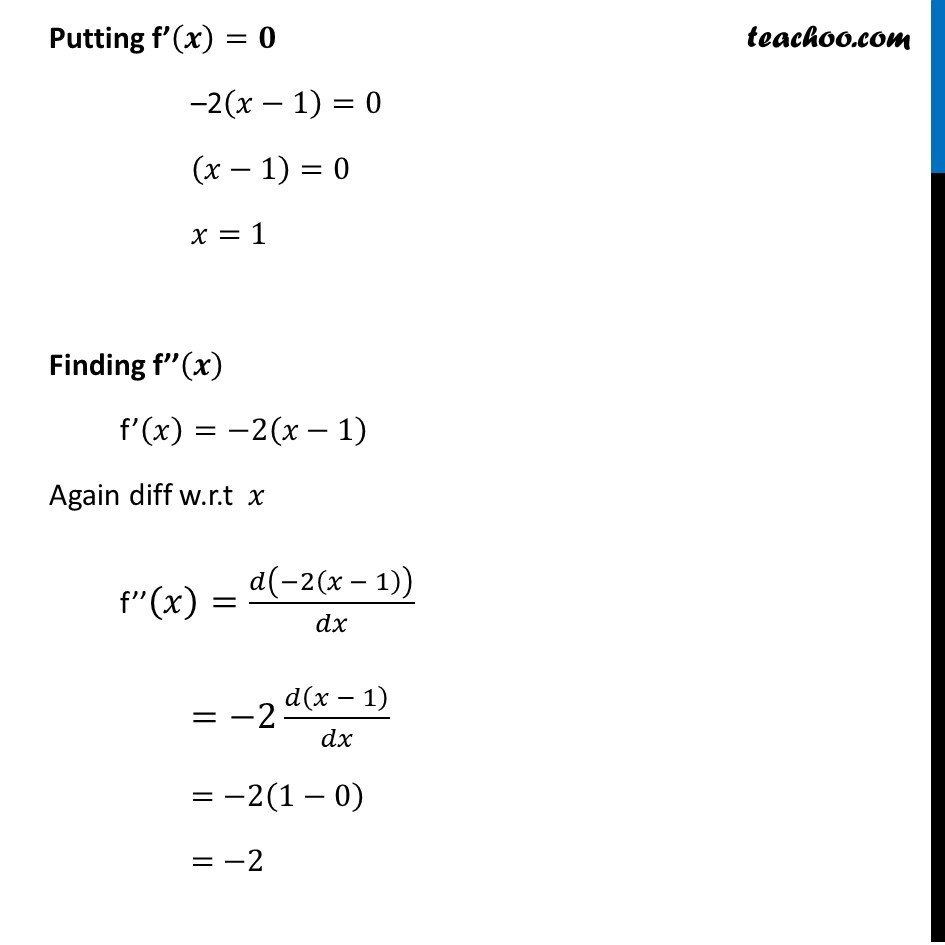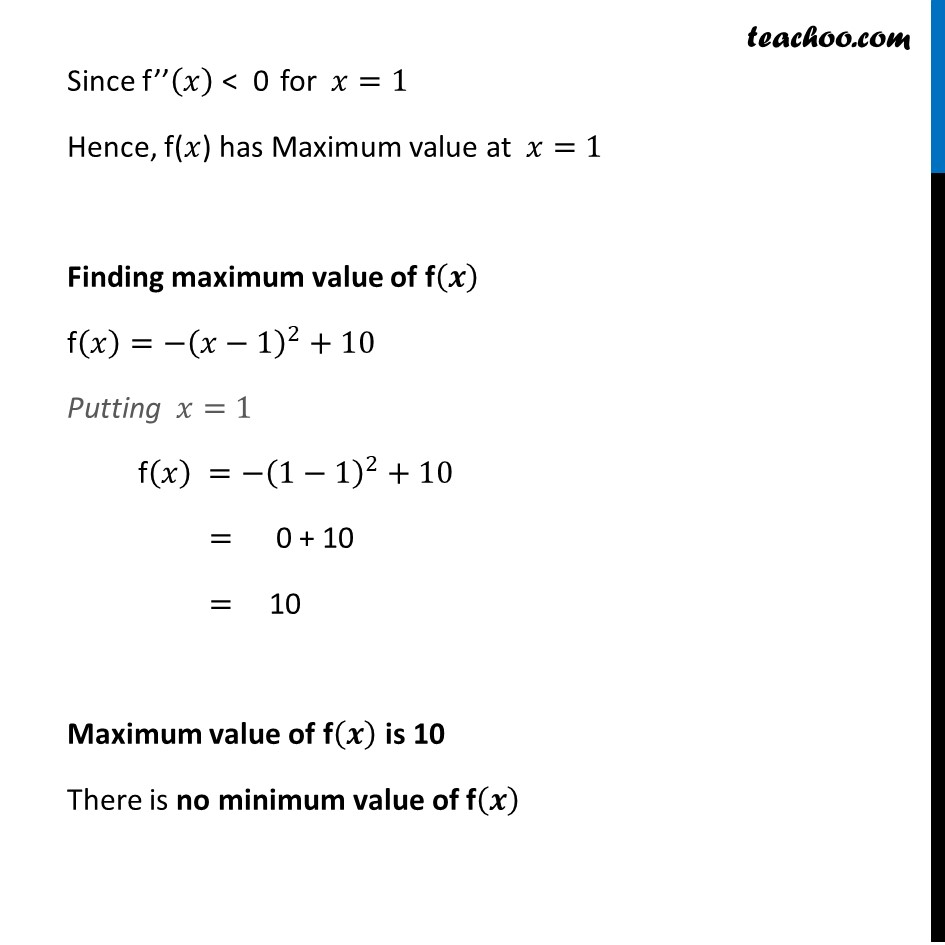Learn in your speed, with individual attention - Teachoo Maths 1-on-1 Class

### Transcript

Ex 6.3,1 (Method 1) Find the maximum and minimum values, if any, of the following functions given by (iii) 𝑓 (𝑥) = –(𝑥 – 1)^2+10 f(𝑥)=−(𝑥−1)^2+10 Finding f’ (x) Diff w.r.t 𝑥 f’(𝑥)=𝑑(−(𝑥−1)^2+10)/𝑑𝑥 f’(𝑥) = –2(𝑥−1)(𝑑(𝑥−1)/𝑑𝑥)+0 f’(𝑥) = –2(𝑥−1)(1−0) + 0 f’(𝑥)=−2(𝑥−1) Putting f’(𝒙)=𝟎 –2(𝑥−1)=0 (𝑥−1)=0 𝑥=1 Hence, 𝑥=1 is point of Maxima & No point of Minima Thus, f(𝑥) has maximum value at 𝑥=1 Putting x=1 in f(x) f(𝑥)=−(𝑥−1)^2+10 f(1)=−(1−1)^2+10 = 0 + 10 = 10 Maximum value of f(𝑥) is 10 There is no minimum value of f(𝒙) Ex 6.3,1 (Method 2) Find the maximum and minimum values, if any, of the following functions given by (iii) 𝑓 (𝑥) = – (𝑥 – 1)^2+10 f(𝑥)=−(𝑥−1)^2+10 Finding f’(x) Diff w.r.t 𝑥 f’(𝑥)=𝑑(−(𝑥−1)^2+10)/𝑑𝑥 f’(𝑥) = –2(𝑥−1)(𝑑(𝑥−1)/𝑑𝑥)+0 f’(𝑥) = –2(𝑥−1)(1−0) + 0 f’(𝑥)=−2(𝑥−1) Putting f’(𝒙)=𝟎 –2(𝑥−1)=0 (𝑥−1)=0 𝑥=1 Finding f’’(𝒙) f’(𝑥)=−2(𝑥−1) Again diff w.r.t 𝑥 f’’(𝑥)=𝑑(−2(𝑥 − 1))/𝑑𝑥 =−2 𝑑(𝑥 − 1)/𝑑𝑥 =−2(1−0) =−2 Since f’’(𝑥) < 0 for 𝑥=1 Hence, f(𝑥) has Maximum value at 𝑥=1 Finding maximum value of f(𝒙) f(𝑥)=−(𝑥−1)^2+10 Putting 𝑥=1 f(𝑥) =−(1−1)^2+10 = 0 + 10 = 10 Maximum value of f(𝒙) is 10 There is no minimum value of f(𝒙)